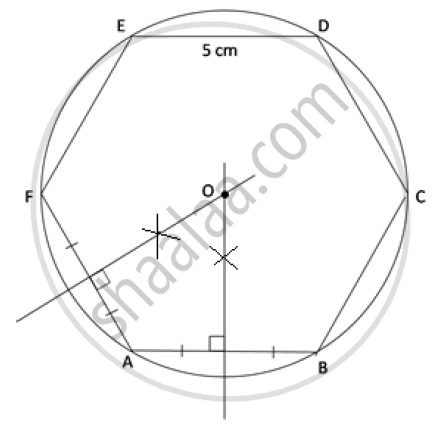Share

# Construct a Regular Hexagon of Side 5 Cm. Construct a Circle Circumscribing the Hexagon. All Traces of Construction Must Be Clearly Shown. - ICSE Class 10 - Mathematics

ConceptCircumscribing and Inscribing a Circle on a Regular Hexagon

#### Question

Construct a regular hexagon of side 5 cm. Construct a circle circumscribing the hexagon. All traces of construction must be clearly shown.

#### Solution

Each interior angle of the regular hexagon = (2n - 4)/n xx90^@ = (2xx6-4)/6 xx 90^2 = 120^@

Steps of construction:

1) Construct the regular hexagon ABCDEF with each side equal to 5 cm

2) Draw the perpendicular bisectors of sides AB and AF and make them intersect each other at point O.

3) With O as the centre and OA as the radius draw a circle which will pass through all the vertices of the regular hexagon ABCDEFIs there an error in this question or solution?

#### APPEARS IN

2014-2015 (March) (with solutions)
Question 4.3 | 4.00 marks

#### Video TutorialsVIEW ALL 

Solution Construct a Regular Hexagon of Side 5 Cm. Construct a Circle Circumscribing the Hexagon. All Traces of Construction Must Be Clearly Shown. Concept: Circumscribing and Inscribing a Circle on a Regular Hexagon.
S